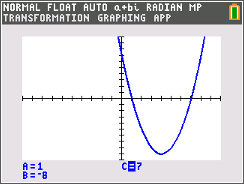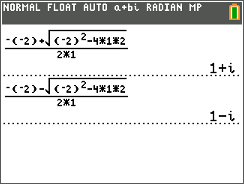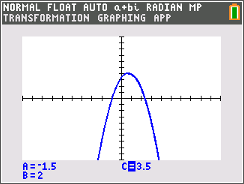# Activities

••• ##### Subject Area

• Math: Precalculus: Polynomial, Power, and Rational Functions
• Math: Precalculus: Functions and Graphs

• ##### Author9-12

45 Minutes

• ##### Device
• TI-84 Plus
• TI-84 Plus Silver Edition
•TI-84 Plus C Silver Edition
•TI-84 Plus CE
• ##### Software

TI Connect™ CE

## Discriminating Against the Zero#### Activity Overview

Students explore when a graph has two zeros, one zero, and no real zeros. They will also determine when a graph has real, rational, irrational, or imaginary roots.

#### Key Steps

•In this activity, students explore the roots or zeros of a quadratic function. They will use the Transformational Graphing application to change the value of b and c in the function f (x) = bx2+bx+c. They will compare the number and type of zeros of the graph to the number under the square root of the quadratic formula. The connections that students make will introduce them to the discriminant.

•After exploring the graph, students will use the quadratic equation to find irrational and imaginary roots. They are to first do it by hand and then check their answers using the graphing calculator.

•The last two parts of the activity can be used as an extension where students are able to change the value of a in the quadratic function. They can also investigate the zeros of the graph when a, b and c are not whole integers.